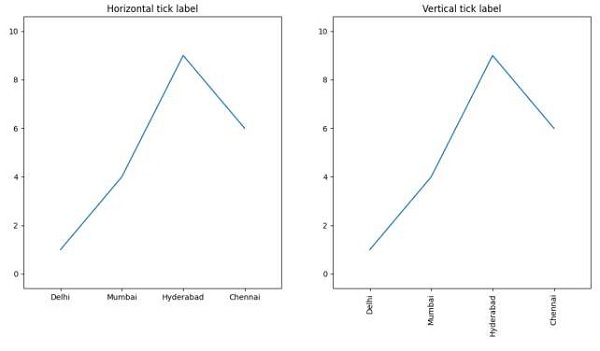# How to rotate X-axis tick labels in Pandas bar plot?

Using plt.xticks(x, labels, rotation='vertical'), we can rotate our tick’s label.

## Steps

• Create two lists, x, and y.

• Create labels with a list of different cities.

• Adjust the subplot layout parameters, where bottom = 0.15.

• Add a subplot to the current figure, where nrow = 1, ncols = 2 and index = 1.

• Plot the line using plt.plot(), using x and y (Step 1).

• Get or set the current tick locations and labels of the X-axis. Pass no arguments to return the current values without modifying them, with x and label data.

• Set or retrieve auto-scaling margins, value is 0.2.

• Set the title of the figure at index 1, the title is "Horizontal tick label".

• Add a subplot to the current figure, where nrow = 1, ncols = 2 and index = 2.

• Plot line using plt.plot() method, using x and y (Step 1).

• Get or set the current tick locations and labels of the X-axis. Pass no arguments to return the current values without modifying them, with x, label data, and rotation = ’vertical’.

• Set or retrieve auto-scaling margins, value is 0.2.

• Set the title of the figure at index 2, the title is "Vertical tick label".

• Use plt.show() to show the figure.

## Example

import matplotlib.pyplot as plt

x = [1, 2, 3, 4]
y = [1, 4, 9, 6]
labels = ['Delhi', 'Mumbai', 'Hyderabad', 'Chennai']

plt.subplot(121)
plt.plot(x, y)
plt.xticks(x, labels)
plt.margins(0.2)
plt.title("Horizontal tick label")

plt.subplot(122)
plt.plot(x, y)
plt.xticks(x, labels, rotation='vertical')
plt.margins(0.2)
plt.title("Vertical tick label")

plt.show()

## Output